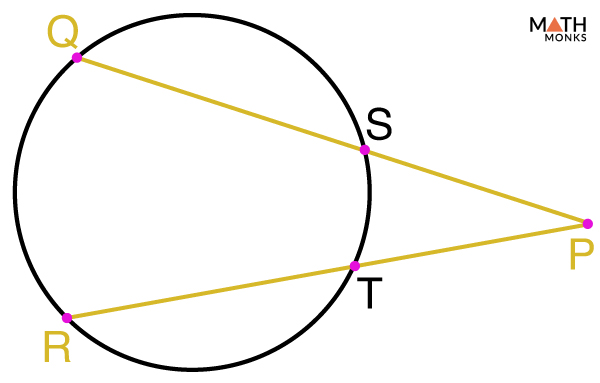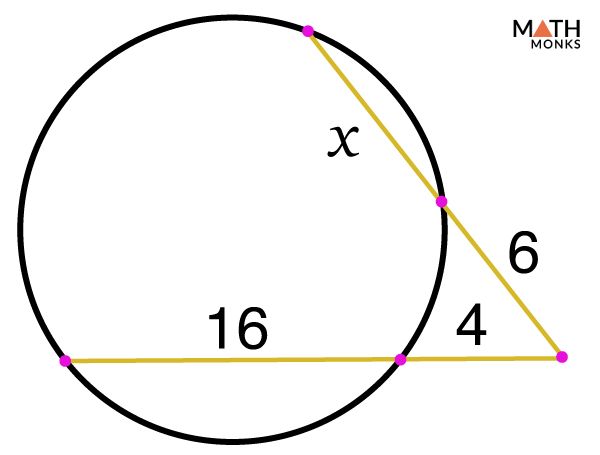# Secant of a Circle

## What is Secant of a Circle

The secant of a circle is a line or line segment that intersects the circle at two points. The term ‘secant’ is obtained from the Latin word ‘secare’ meaning to ‘to cut’. It can also be explained as the extension of the chord of a circle that goes outside the circle.

In the circle given below, ‘PQ’ is the secant.

## Relation between Secant, Chord and Tangent of a Circle

As discussed above, a straight line or line segment that intersects a circle at two points is a secant. A chord, on the other hand, is a line segment that joins two distinct points of the circle. In the circle shown above, AB is the chord, which is a part of the secant PQ. Thus, a chord that joins two points on the circumference of a circle, if extended on both sides becomes a secant.  If a chord passes through the center of the circle, then it is the diameter.

Finally, a tangent is known to be a line or a line segment that intersects a circle at exactly one point.

## Properties of a Secant with Formulas

When two secants or a tangent and a secant are drawn, they can intersect in 3 ways forming 4 relationships. The relationship formed by such intersection is given below:

Case 1: When two secants intersect outside the circle

When two secants of a circle intersect each other at a point outside the circle then the product of the length of one whole secant segment and its external segment is equal to the product of the other whole secant segment and its external segment. This is also known as the intersecting secants theorem.

Case 2: When two secants intersect inside the circle

If two secants intersect inside the circle, then the product of each of the parts within a segment is equal to the product of each of the parts of the other segment.

Case 3: When two secants or a secant and a tangent intersect outside the circle

If two secants or a secant and a tangent intersect outside the circle then the angle formed is half the positive difference of the measure of the intercepted arcs.

a) When two secants intersect outside the circle

b) When a secant and a tangent intersect outside the circle

Case 4: When a secant and a tangent intersect outside the circle

If a secant and a tangent intersect outside the circle, then the square of the length of the tangent is equal to the product of the length of one whole secant segment and its external segment.

### Derivation

The above relationship in ‘Case-4’ can be used to find the secant of a circle if the length of the intersecting tangent and the length of the external segment of the secant are known. The formula is derived below:

According to ‘Case – 4’

(Length of the tangent)2 = Length of the secant × Length of the External Segment  of the Secant, here SQ = length of the tangent, QR = Length of the secant, QT = Length of the External Segment  of the Secant

Mathematically,

SQ2 = QR × QT

QR = SQ2/ QT

Thus,

Length of the secant = (length of the tangent)2/Length of the External Segment  of the Secant

## Solved ExamplesIn the circle shown, if PS =10, SQ =17, PT=9, find the length of TR.

Solution:

As we know,
According to the Intersecting Secants Theorem
PQ × PS = PR × PT
(PS + SQ) × PS = (PT + TR) × PT, here PS =10, SQ =17, PT=9
(10 + 17) × 10 = (9 + TR) × 9
270 = 81 + 9TR
189 = 9TR
TR = 21In the circle given alongside, mPT =100°, mRP =120°, and mTS =100.

Solution:

The given figure is an example of two secants intersecting inside the circle. Thus, the angle formed is equal to half the positive difference of the measure of the intercepted arcs.
Mathematically,
m∠RQS = (mPT – mRS)/2, here mPT =100°
To find mRS = 360° – (mPT  + mRP + mTS) (since a full circle is 360°)
=> mRS = 360° – (100° + 120° + 100°)
=> mRS = 40°
Now, to solve the measure of ∠RQS
m∠RQS = (mPT – mRS)/2
=> m∠RQS = (100° – 40°)/2
=> m∠RQS = 30°Find x in the given figure.

Solution:

As we know from the ‘Two Secants Segments Theorem’
=> 6(6 + x) = 4(4 + 16)
=> 36 + 6x = 16 + 64
=> 6x = 44
=> x = 7.33

1.b.r muddapur says:
•staff says: